# Robin's Blog

## Ten Little IDL programs in Python

I recently saw Michael Galloy’s post at http://michaelgalloy.com/2016/02/18/ten-little-idl-programs.html, showing some short (less than ten lines long) programs in IDL. I used to do a lot of programming in IDL, but have switched almost all of my work to Python now – and was intrigued to see what the code looked like in Python.

I can’t guarantee that all of my Python code here will give exactly the same answer as the IDL code – but the code should accomplish the same aim. I’ve included the IDL code that Michael provided, and for each example I provide a few comments about the differences between the Python and IDL code. I haven’t shown the output of the IDL examples in the notebook (yes, I know I can run IDL through the Jupyter Notebook, but I don’t have that set up on this machine.

Firstly, we import the various modules that we need for Python. This is rather different to IDL, where all of the functionality for these programs is built in – but is not necessarily a disadvantage, as it allows an easier separation of functionality, allowing you to only include the functions you need. I’m going to ‘cheat’ slightly, and not count these import lines in the number of lines of code used below – which I think is fair.

%matplotlib inline
import os
import numpy as np
import pandas as pd
from matplotlib.pyplot import *
from PIL import Image
from skimage.filters import sobel
from skimage.feature import canny

It is also worth noting that counting the number of lines of a bit of Python code is rather arbitrary – because although whitespace is syntactically important, you can still often combine multiple lines into one line. For example:

a = 5; b = 10; print(a+b)

15

Here I’m going to keep to writing Python in a sensible, relatively standard (hopefully PEP8 compliant) way.

### 1 line: output, calling a procedure:

#### IDL

print, 'Hello, world!'


#### Python

print("Hello, world!")

Hello, world!

These are almost exactly the same…not much to see here!

### 2 lines: assignment, calling a function, system variables, array operations, keywords:

#### IDL

x = findgen(360) * !dtor
plot, x, sin(x), xstyle=9, ystyle=8


#### Python

x = np.radians(np.arange(360))
plot(x, np.sin(x), 'r-')

[<matplotlib.lines.Line2D at 0x117aa4588>]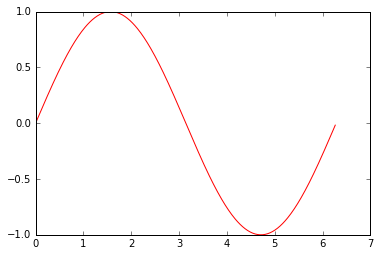This is fairly similar, the major differences being the use of the np. prefix on various functions as they are part of the numpy library (this can be avoided by importing numpy as from numpy import *, but that is not recommended). The only other real differences are the use of a function to convert from degrees to radians, rather than a constant conversion factor, and the differences in the name of the function that produces an array containing a range of values – I personally found findgen always made me thinking of FINDing something, rather than Floating INDex GENeration, but that’s just me!

### 3 lines: input, output format codes:

#### IDL

name = ''
print, name, format='("Hello, ", A, "!")'


#### Python

name = input('What is your name? ')
print("Hello {name}!".format(name=name))

What is your name? Robin
Hello Robin!

This is the first example where the lengths differ – and Python is very slightly shorter. The only reason for this is that IDL requires you to initialise the name variable before you can read into it, whereas Python does not. I prefer the way that the formatting of the string works in Python – although this is but one of multiple ways of doing it in Python. For reference, you could also do any of the following:

print("Hello %s!" % (name))
print("Hello " + name + "!")


### 5 lines: filename handling, reading images, variable metadata, boolean keywords, displaying image:

#### IDL

filename = filepath('people.jpg', subdir=['examples', 'data'])
dim = size(im, /dimensions)
window, xsize=dim, ysize=dim, /free, title=file_basename(filename)
tv, im, true=1


#### Python

filename = os.path.join('examples', 'data', 'flowers.tif')
im = Image.open(filename)
imshow(im)
title(os.path.basename(filename))

<matplotlib.text.Text at 0x118249ef0>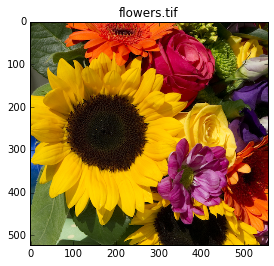This example is also slightly shorter in Python, mainly because we don’t have to create the display window manually, and therefore we don’t need to find out the size of the image before-hand. On the other hand, Python has no way to set the title of a plot in the call to the plotting function (in this case imshow, which I personally think is a more understandable name than tv), which adds an extra line.

### 6 lines: logical unit numbers, read binary data, contour plots, line continuation:

#### IDL

convec = bytarr(248, 248)
openr, lun, file_which('convec.dat'), /get_lun
free_lun, lun
window, xsize=500, ysize=500, /free
contour, convec, xstyle=1, ystyle=1, $levels=(max(convec) - min(convec)) * findgen(10) / 9. + min(convec)  #### Python convec = np.fromfile('convec.dat', dtype=np.byte) convec = convec.reshape((248, 248)) contour(convec)  <matplotlib.contour.QuadContourSet at 0x1182a4c50>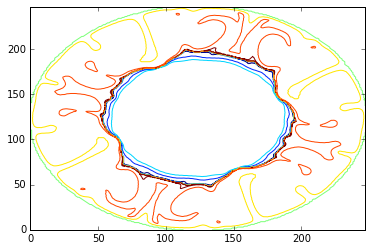This is also shorter, although I must admit that I haven’t configured the contour levels manually as was done in the IDL code – as I often find I don’t need to that. Again, you can see that we don’t need to create the array before we read in the file, and we don’t have to deal with all of the opening, reading and closing of the file as the np.fromfile function does all of that for us. (If we did want to work at a lower level then we could – using functions like open and close). I’ve also shown a line continuation in Python, which in many circumstances works with no explicit ‘continuation characters’ – even though it wasn’t really needed in this situation. ### 7 lines (contributed by Mark Piper): query image, image processing, automatic positioning of images: #### IDL file = file_which('moon_landing.png') !null = query_image(file, info) astronaut = read_image(file) window, /free, xsize=3 * info.dimensions, ysize=info.dimensions tv, astronaut, 0 tvscl, sobel(astronaut), 1 tvscl, canny(astronaut), 2  #### Python im = Image.open('examples/data/flowers.tif') im = np.array(im)[:, :, 0] figure(figsize=(14, 10)) subplot(131) imshow(im) subplot(132) imshow(canny(im), interpolation='nearest', cmap=cm.gray) subplot(133) imshow(sobel(im), interpolation='nearest', cmap=cm.gray)  <matplotlib.image.AxesImage at 0x1193adc18>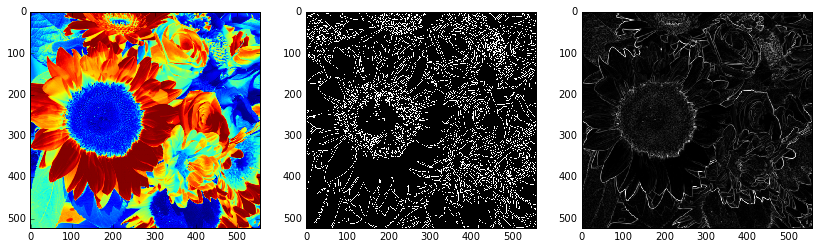Here the Python version is longer than the IDL version – although the majority of this length comes from the subplot commands which are used to combine multiple plots into one window (or one output image). Apart from that, the majority of the code is very similar – albeit with some extra parameters for the python imshow command to force nearest-neighbour interpolation and a gray-scale colormap (though these can easily be configured to be the defaults). ### 8 lines: writing a function, compile_opt statement, if statements, for loops: #### IDL .compile function mg_fibonacci, x compile_opt strictarr if (x eq 0) then return, 0 if (x eq 1) then return, 1 return, mg_fibonacci(x - 1) + mg_fibonacci(x - 2) end for i = 0L, 20L do print, i, mg_fibonacci(i)  #### Python def mg_fibonacci(x): if x == 0: return 0 if x == 1: return x else: return mg_fibonacci(x - 1) + mg_fibonacci(x - 2) for i in range(10): # Only 10 lines of output to keep the blog post reasonably short! print(i, mg_fibonacci(i))  0 0 1 1 2 1 3 2 4 3 5 5 6 8 7 13 8 21 9 34  The code here is almost the same length (9 lines for Python and 8 for IDL), even though the Python code looks a lot more ‘spacious’. This is mainly because we don’t need the .compile or compile_opt lines in Python. Apart from that, the code is very similar with the main differences being Python’s use of syntactic whitespace and use of ‘proper’ equals signs rather than IDL’s eq (and gt, lt etc). ### 9 lines (contributed by Mark Piper): array generation, FFTs, line plots, multiple plots/window, query for screen size: #### IDL x = (2.0 * !pi) * findgen(100) / 100 y = sin(3.0 * x) + cos(12.0 * x) + cos(25.2 * x) magspec_y = abs(fft(y)) ss = get_screen_size() window, /free, xsize=0.4 * ss, ysize=0.25 * ss !p.multi = [0,2,1] plot, x, y, xtitle='sample number', ytitle='value', title='Series', xstyle=1 plot, magspec_y, xtitle='mode', ytitle='spectral density',$
title='Magnitude Spectrum', xrange=[0, n_elements(x) / 2], xstyle=1
!p.multi = 0


#### Python

x = (2.0 * np.pi) * np.arange(100) / 100.0
y = np.sin(3.0 * x) + np.cos(12.0 * x) + np.cos(25.2 * x)

magspec_y = abs(np.fft.fft(y))

figure(figsize=(12, 4))
subplot(121)
plot(x, y)
xlabel('sample number')
ylabel('value')
title('Series')
subplot(122)
plot(magspec_y)
xlabel('mode')
ylabel('spectral density')
title('Magnitude Spectrum')

<matplotlib.text.Text at 0x11a380e80>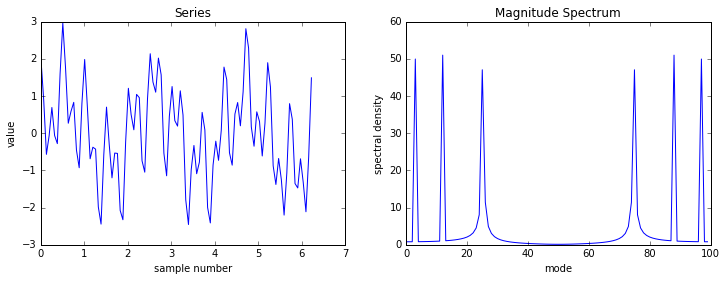The Python code is a lot longer here, but that is mainly due to Python requiring a separate function call to set each piece of text on a plot (the title, x-axis label, y-axis label etc). Apart from that there aren’t many differences beyond those already discussed above.

### 10 lines: maps, read ASCII file, indexed color, structures:

#### IDL

header = strarr(5)
data = replicate({loc:fltarr(2), elev:0, temp:0, dew:0, wspd:0, wdir:0}, 15)
openr, lun, file_which('ascii.txt'), /get_lun
free_lun, lun
device, decomposed=0
map_set, limit=[min(data.loc[1, *], max=maxlat), $min(data.loc[0, *], max=maxlon), maxlat, maxlon], /mercator, /usa plots, data.loc[0, *], data.loc[1, *], psym=4, color=bytscl(data.temp),$
symsize=2., thick=2


#### Python

I’m going to go through the Python code in a few bits for this one…
Firstly, reading CSVs in Python is really easy using the pandas library. The first six lines of IDL code can be replaced with this single function call:

df = pd.read_csv('/Users/robin/ascii.txt', skiprows=5,
names=['lon', 'lat', 'elev', 'temp', 'dew', 'wspd', 'wdir'])

And you can print out the DataFrame and check that the CSV has loaded properly:

df

lon lat elev temp dew wspd wdir
0 -156.9500 20.7833 399 68 64 10 60
1 -116.9667 33.9333 692 77 50 8 270
2 -104.2545 32.3340 1003 87 50 10 340
3 -114.5225 37.6073 1333 66 35 0 0
4 -106.9418 47.3222 811 68 57 8 140
5 -94.7500 31.2335 90 89 73 10 250
6 -73.6063 43.3362 100 75 64 3 180
7 -117.1765 32.7335 4 64 62 5 200
8 -116.0930 44.8833 1530 55 51 0 0
9 -106.3722 31.8067 1206 82 57 9 10
10 -93.2237 30.1215 4 87 77 7 260
11 -109.6347 32.8543 968 80 46 0 0
12 -76.0225 43.9867 99 75 66 7 190
13 -93.1535 36.2597 415 86 71 10 310
14 -118.7213 34.7395 1378 71 46 5 200
Unfortunately the code for actually plotting the map is a bit more complicated, but it does lead to a nice looking map. Basically, the code below creates a map with a specified extent: this is controlled by the keyword arguments called things like llcrnrlat. I usually find that Python has more understandable names than IDL, but in this case they’re pretty awful: this stands for “lower-left corner latitude”.
Once we’ve created the map, and assigned it to the variable m, we use various methods to display things on the map. Note how we can use the column names of the DataFrame in the scatter call – far nicer than using column indexes (as it also works if you add new columns!). If you un-comment the m.shadedrelief() line then you even get a lovely shaded relief background…

from mpl_toolkits.basemap import Basemap

figure(figsize=(15,20))
m = Basemap(llcrnrlat=df.lat.min()-5, llcrnrlon=df.lon.min()-5,
urcrnrlat=df.lat.max()+5, urcrnrlon=df.lon.max()+5,
resolution='l')

m.drawmapboundary()
m.drawcoastlines()
xs, ys = m(df.lon, df.lat)

m.scatter(df.lon, df.lat, c=df.temp,
marker='o', s=100, cmap=cm.RdBu_r, latlon=True)
m.colorbar(label='Temperature')

<matplotlib.colorbar.Colorbar at 0x11c773278>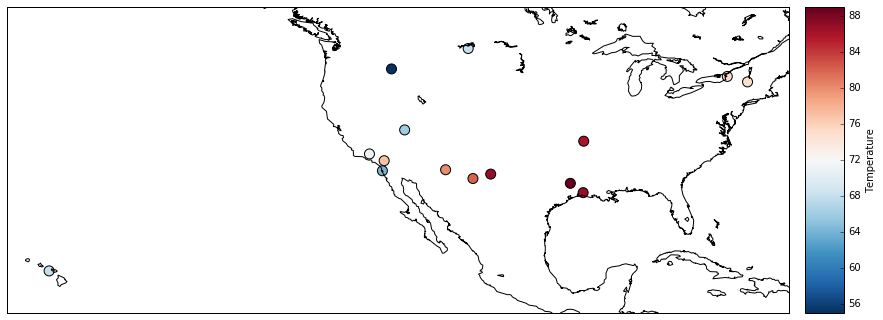Just as a little ‘show off’ at the end of this comparison, I wanted to show how you can nice interactive maps in Python. I haven’t gone into any of the advanced features of the folium library here – but even just these few lines of code allow you to interactively move around and see where the points are located: and it is fairly easy to add colours, popups and so on.

import folium

map_osm = folium.Map(location=[32,-104], zoom_start=3)
for i, row in df.iterrows():
map_osm


### Summary

So, what has this shown?
Well, I don’t want to get in to a full IDL vs Python war…but I will try and make a few summary statements:

• Sometimes tasks can be achieved in fewer lines of code in IDL, sometimes in Python – but overall, the number of lines of code doesn’t really matter: it’s far more important to have clear, easily understandable code.
• The majority of the tasks are accomplished in a very similar way in IDL and Python – and with a bit of time most experienced programmers could work out what code in either language is doing.
• A number of operations can be achieved in a simpler way using Python – for example, reading files (particularly CSV files) and displaying plots – as they don’t require the extra boilerplate code that IDL requires (to do things like get the screen size, open a display window, create an empty array to read data into etc).
• Most IDL plotting functions take arguments allowing you to set things like the x-axis label or title of the plot in the same function that you use to plot the data – whereas Python requires the use of separate functions like xlabel and title.
• I tend to find that Python has more sensible names for functions (things like arange rather than findgen and imshow rather than tv) – but that is probably down to personal taste.
• In my opinion, Python’s plots look better by default than IDLs plots – and if you don’t like the standard matplotlib style then they can be changed relatively easily. I’ve always struggled to get IDL plots looking really nice – but that may just be my lack of expertise.
• IDL has a huge amount of functionality ‘baked-in’ to the language by default, whereas Python provides lots of functionality through external libraries. Many of the actual functions are almost exactly equivalent – however there a number of disadvantages of the library-based approach, including issues with installing and updating libraries, lack of support for some libraries, trying to choose the best library to use, and the extra ‘clutter’ that comes from having to import libraries and use prefixes like np..

Overall though, most things can be accomplished in either language. I prefer Python, and do nearly all of my programming in Python these days: but it’s good to know that I can still drop back in to IDL if I need to – for example, when interfacing with ENVI.

The Jupyter Notebook used to create this post is available to download here.

Categorised as: Programming, Python

1.Marcel Stimberg says:

As you said, line-counting is of course a bit silly, but if you want to reduce the number of lines for the “9 lines” Python example, try replacing the plotting code with:

f, (ax1, ax2) = subplots(1, 2, figsize=(12, 4))
ax1.plot(x, y)
ax1.set(xlabel=’sample number’, ylabel=’values’, title=’Series’)
ax2.plot(magspec_y)
ax2.set(xlabel=’mode’, ylabel=’spectral density’, title=’Magnitude Spectrum’)

2.Robin Wilson says:

Thank you – I knew about the subplots function (I end up accidentally running it rather than the subplot function!), but I didn’t know about the axis.set function – that’s good to know!

3.Luke says:

An interesting post as I have just started porting some of my IDL scripts to Python.

It’s worth noting since version 7.1 of IDL, a CSV can be really easily read into a structure variable:

The new graphics functions introduced in version 8.0 e.g. plot() are also far superior to the old direct graphics routines, and make it much easier to produce nice looking figures.

4.Robin Wilson says:

Interesting – thanks Luke. I’d never really got into the ‘new graphics’ in IDL, as I think when they were first released (early in my PhD) they were fairly limited, but it sounds like they’re pretty good now!

5. […] wrote a blog post comparing a set of ‘Ten Little Programs’ in IDL with equivalents in Python, which […]

6. […] wrote a blog post comparing a set of ‘Ten Little Programs’ in IDL with equivalents in Python, which […]

7.Derek says:

Re Fibonacci; you can write the Python in 5 lines if you wanted, in a grammatically-legal way:

def mg_fibonacci(x):
if x == 0: return 0
if x == 1: return x
return mg_fibonacci(x – 1) + mg_fibonacci(x – 2)
for i in range(10): print(i, mg_fibonacci(i))

The point is, Python has the core philosophy that favors readability over “compact, clever, code golf”.

But I still think you could drop the ‘else:’ line and the code would be just as readable 🙂

8.Timothy Henry says:

Disingenuous at best. Not mentioned are the number of lines necessary to do anything in Python. Import this, that and the other. Once a plot is made. you have to recreate it to modify it in Python. compile_opt is unnecessary in the 8-line program. In IDL, it is interactive. Development is more straight forward and
once developed, as with python, the bottom line isn’t the number of lines it takes.

9.Timothy Henry says:

Function mg_fibonacci, x
If x LE 1 Then Return, x \$
Else Return, mg_fibonacci(x-1)+mg_fibonacci(x-2)
End

10.Greg says:

Function mg_fibonacci, x
Return,(x LE 1)?x:mg_fibonacci(x-1)+mg_fibonacci(x-2)
End